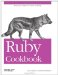# Building Up a Hash Using Injection

Problem

You want to create a hash from the values in an array.

Solution

As seen in Recipe 4.8, the most straightforward way to solve this kind of problem is to use Enumerable#inject. The inject method takes one parameter (the object to build up, in this case a hash), and a block specifying the action to take on each item. The block takes two parameters: the object being built up (the hash), and one of the items from the array.

Here's a straightforward use of inject to build a hash out of an array of key-value pairs:

```	collection = [ [1, 'one'], [2, 'two'], [3, 'three'],
[4, 'four'], [5, 'five']
]

collection.inject({}) do |hash, value|
hash[value.first] = value.last
hash
end
# => {5=>"five", 1=>"one", 2=>"two", 3=>"three", 4=>"four"}
```

Discussion

Why is there that somewhat incongrous expression hash at the end of the inject block above? Because the next time it calls the block, inject uses the value it got from the block the last time it called the block. When you're using inject to build a data structure, the last line of code in the block should evaluate to the object you're building up: in this case, our hash.

This is probably the most common inject-related gotcha. Here's some code that doesn't work:

```	collection.dup.inject({}) { |hash, value| hash[value.first] = value.last }
# IndexError: index 3 out of string
```

Why doesn't this work? Because hash assignment returns the assigned value, not the hash.

```	Hash.new["key"] = "some value" # => "some value"
```

In the broken example above, when inject calls the code block for the second and subsequent times, it does not pass the hash as the code block's first argument. It passes in the last value to be assigned to the hash. In this case, that's a string (maybe "one" or "four"). The hash has been lost forever, and the inject block crashes when it tries to treat a string as a hash.

Hash#update can be used like hash assignment, except it returns the hash instead of the assigned value (and it's slower). So this code will work:

```	collection.inject({}) do |hash, value|
hash.update value.first => value.last
end
# => {5=>"five", 1=>"ontwo", 2=>"two", 3=>"three", 4=>"four"}
```

Ryan Carver came up with a more sophisticated way of building a hash out of an array: define a general method for all arrays called to_h.

```	class Array
def to_h(default=nil)
Hash[ *inject([]) { |a, value| a.push value, default || yield(value) } ]
end
end
```

The magic of this method is that you can provide a code block to customize how keys in the array are mapped to values.

```	a = [1, 2, 3]

a.to_h(true)
# => {1=>true, 2=>true, 3=>true}

a.to_h { |value| [value * -1, value * 2] }
# => {1=>[-1, 2], 2=>[-2, 4], 3=>[-3, 6]}
```

References

• Recipe 5.3, "Adding Elements to a Hash"
• Recipe 5.12, "Building a Histogram"
• The original definition of Array#to_h:(http://fivesevensix.com/posts/2005/05/20/array-to_h)Ruby Cookbook (Cookbooks (OReilly))
ISBN: 0596523696
EAN: 2147483647
Year: N/A
Pages: 399